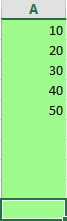0
Thanks

A few words of thanks would be greatly appreciated.

# Excel VBA: Loops procedure explained.## Excel VBA Loops

Loops are one of the most basic and powerful programming tools in VBA, and used across most programming languages. Loops are used to repeat a block of code as many times as required, until a given condition remains true or a specific point (or value) is reached, after which the the next section of code is executed. A loop enables you to write a few simple lines of code and achieve a far more significant output, just by repetition.

There are three basic kinds of VBA Loops (subdivided into 6 loops as below):

## For Loop

### For ... Next Statements

repeats a block of code a specific number of times.
the following example will show a MsgBox for 5 times, and display the number.

```Sub F_Next_loop()

Dim i As Integer

For i = 1 To 5
MsgBox i
Next i

End Sub```

### For Each ... Next Statements

The For Each ... Next Loop repeats a block of code for each object in a group. It repeats execution of a block of code, for each element of a collection. The loop stops when all the elements in the collection have been covered, and execution moves to the section of code immediately following the Next statement.

```Sub F_each_loop()

Dim Cell As Range
For Each Cell In ActiveSheet.Range("A1:A10")
Cell.Interior.Color = RGB(160, 251, 142)
Next Cell

End Sub````The Exit For statement will immediately stop execution of the existing loop and execute the section of code immediately following the Next statement,`

## Do While Loop

repeats a block of code indefinitely while the specified condition continues to be met and evaluated to True, and stops when the condition turns False. The condition can be tested either at the start or at the end of the Loop.

### Do While ... Loop Statements

test the condition at the start,

```Sub do_While()

Dim i As Integer
i = 1
Do While Cells(i, 1).Value <> ""
MsgBox i
i = i + 1
Loop
MsgBox i

End Sub```

### Do ... Loop While Statements

test the condition at the end of the Loop

```Sub do_While()

Dim i As Integer
i = 1
Do
MsgBox i
i = i + 1
Loop While Cells(i, 1).Value <> ""
MsgBox i

End Sub```
`The Exit Do statement will immediately stop execution of the existing loop and execute the section of code immediately following the Next statement,`

## Do Until Loop

repeats a block of code indefinitely until the condition is met and evaluates to True. The condition can be tested either at the start or at the end of the Loop

### Do Until ... Loop Statements

test the condition at the start,

```Sub do_Until()

Dim i As Integer
i = 1
Do Until Not IsEmpty(Cells(i, 1))
Cells(i, 1).Interior.Color = RGB(255, 0, 0)
i = i + 1
Loop

End Sub```

### Do ... Loop Until Statements

test the condition at the end of the Loop.

```Sub do_Until()

Dim i As Integer
i = 1
Do
Cells(i, 1).Interior.Color = RGB(255, 0, 0)
i = i + 1
Loop Until Not IsEmpty(Cells(i, 1))

End Sub
```
0
Thanks

A few words of thanks would be greatly appreciated.